# Mutable List Structure

## Mutating a Pair

In Unit 2, we used pairs as the foundation of the data structures that store data. We have seen that we are now walking in a realm where it's possible to mutate data. There will also be times when we want to mutate what is stored in our data structures.

(define x (cons 1 2))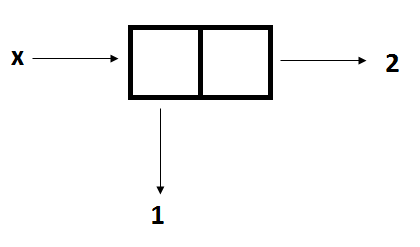### set-car!

Let the car of x represent the number of times I fall down the stairs and the cdr of x represent the number of times I went to the wrong bathroom. I fell down a flight of stairs just now, so I should update the car of x to 2. How can we achieve this without creating a new pair? Scheme allows us to set! the value of some item using the following function:

(set-car! x 2)


As the name suggests, set-car! takes in a pair and a value, and changes its car to point to the specified value of the second argument.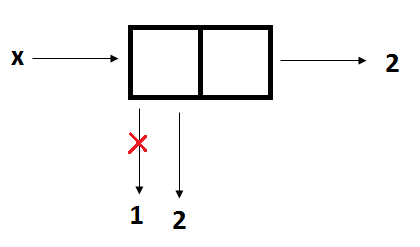The general form is

(set-car! <pair> <value>)


### set-cdr!

As you might expect, Scheme also provides us with the function set-cdr!, which takes in a pair and a value, and changes the pointer of the pair's cdr to point to the value. Going with the previous example, calling

(set-cdr! x 3)


will change the pair as shown below.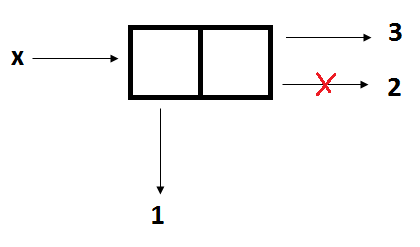The general syntax is

(set-cdr! <pair> <value>)


## Changing Pointers

Let us see how set-car! and set-cdr! work with more complicated lists. We are given the following pairs:

(define x (cons (list a b) (list c d)))
(define y (list e f))The next few calls on set-car! and set-cdr! are independent and will be based on this original configuration. For the next few questions, drawing the box-pointer is highly recommended.

### Example 1

The effect of calling

(set-car! x y)


to the original configuration will give the resulting box-and-pointer diagram:The call changes the car-pointer on x, which initially points to (list a b), to wherever y is pointing: (list e f). What happens to the list with a and b? Nothing actually happens to it, but since it has no pointers that reference it, the list is no longer reachable.

In Example 1 above, what does x print?.
Let's say we have the original configuration from Example 1, and now we decided to call the following expression:
(set-car! (car x) 'z)
What does y print?

### Example 2

From the original configuration, we now call

(define z (cons y (cdr x)))


This gives us the following box-and-pointer diagram:• x will print ((a b) c d)

• y will print (e f)

• z will print ((e f) c d)

From the configuration shown in Example 2, we now decide to call
(set-cdr! (cdr z) nil)
What does x print now?
From the configuration shown in Example 2, what should we call so that z will return ((e f) b)?

## Creating New Pairs

Using set-car! and set-cdr! modifies existing pairs. Procedures like cons and list on the other hand, creates a new pair. What about append? Does it 'merge' two lists by changing the cdr pointer of one of them? Remember the definition of append we have been using:

(define (append x y)
(if (null? x)
y
(cons (car x) (append (cdr x) y))))


append forms a new list by cons-ing elements of x and y. This tells us that append returns a new list.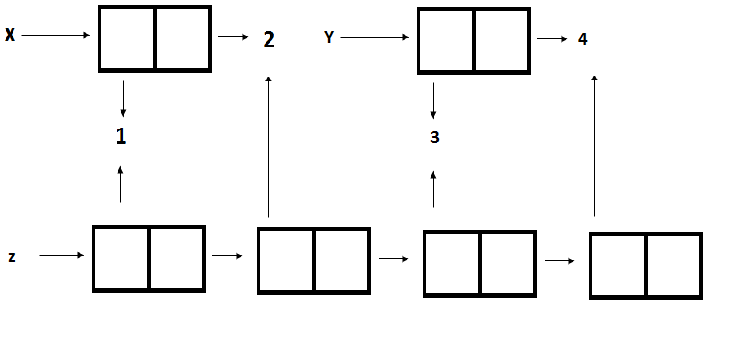What will the following piece of code print when entered into STk? Take an educated guess, then try it out in the interpreter. > (define a (list 1 2 3 4 5)) a > (set-cdr! (cdr a) (cdddr a)) okay > a
The procedure append! is similar to append, but it is a mutator rather than a constructor. It appends the lists by splicing them together, modifying the final pair of x so that its cdr is now y. (It is an error to call append! with an empty x.) For example,
(define (append! x y)
(set-cdr! (last-pair x) y)
x)
The last-pair procedure accepts a list and returns the last pair of the list:
 (define (last-pair x)
(if (null? (cdr x))
x
(last-pair (cdr x))))
Now, let's take a look at this piece of code:
> (define x (list 'a 'b))
x
> (define y (list 'c 'd))
y
> (define z (append x y))
z
> z
(a b c d)
What does (cdr x) return? Try it out by yourself before putting it into STk. Now, take the following call to append!
> (define w (append! x y))
w
> w
(a b c d)
What does (cdr x) return now?

## Sharing and Identity

The previous exercises raises a big sign that knowing when a pair is shared and created is important. In the code above, x and y refer to the same pairs, while z makes a different pair with the same elements.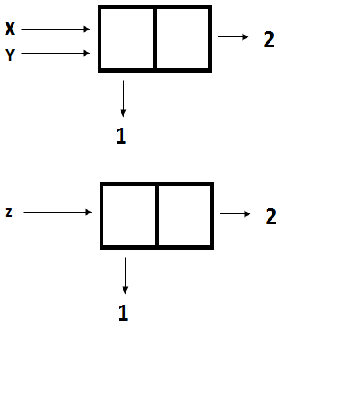(define x (cons 1 2))
(define y x)
(define z (cons 1 2))


Remember the equal? predicate? It can check if two pairs contain the same elements.

(equal? x y), (equal? x z), (equal? y z) all return #t, because they all hold the same elements at the same place. Is it possible to differentiate that x and z point to different structures? Yes! Scheme has the eq? predicate which takes 2 arguments and checks if the 2 arguments refer to the same pair.

> (eq? x y)
#t
> (eq? x z)
#f
> (eq? y z)
#f


## Takeaways

set-car! and set-cdr! change the respective car and cdr pointers. Procedures like cons, list, and append create new pairs. Knowing which pairs are shared between different lists is crucial to determining whether mutating one will influence the other. Drawing box-and-pointer diagrams will be very helpful.

9 - Mutable Data and Vectors